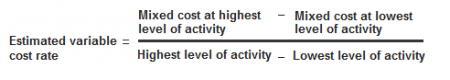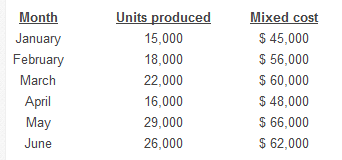Accounting

# What is High-low point method?

High-low point method is often a technique used in order to divide a merged cost into their variable and set components.
Sometimes it will be important to determine your fixed and variable the different parts of a mixed expense figure. Several techniques are used for this function such as scatter-graph process, least squares process and high-low place method. On this page I am going to explain using high-low point method.

Under this process, an estimated adjustable cost rate is actually calculated first when using the highest and most affordable activity levels as well as mixed costs regarding them. This estimated adjustable cost rate can be used to calculate full estimated variable cost within the mixed cost numbers at highest as well as lowest activity quantities. The estimated adjustable cost is then subtracted from your total mixed expense figures at highest and lowest activity levels to find the fixed cost aspect.

To make the procedure quick and simple to understand, we can easily divide the calculations into your following three methods.

Step 1 – calculation of variable cost rate:

The first step in high-low point method is to calculate an estimated variable cost rate. This rate is calculated by using the following formula:Step 2 – calculation of variable cost component:

After calculating estimated variable cost rate, the second step is to calculate the total estimated variable cost at highest and lowest activity levels. It is calculated by multiplying the estimated variable cost rate (calculated in step 1) by highest and lowest activity levels.

Estimated Total Variable Cost = Estimated Variable Cost Rate * Highest or Lowest Level of Activity

Step 3 – calculation of fixed cost component:

The third and final step in high-low point method is to find out the fixed cost component of the total mixed cost. It is calculated by subtracting the estimated variable cost (calculated in step 2) from the total mixed cost figure.

Fixed Cost + Total Mixed Cost – Estimated Total Variable Cost

Example

The Western company presents the production and cost data for the first six months of the 2015.Required: Determine the estimated variable cost rate and fixed cost using high-low point method.

Solution

Variable cost rate

(66,000 – 45,000)/(29,000 – 15,000)

= \$21000/14,000

= \$1.5 per unit

Variable cost:

• Highest activity level (May): 29,000 units × \$1.5 = \$43,500
• Lowest activity level (January): 15,000 × \$1.5 = \$22,500

Fixed cost:

• Highest activity level (May): \$66,000 – \$43,500 = \$22,500
• Lowest activity level (January): \$45,000 – \$22,500 = \$22,500